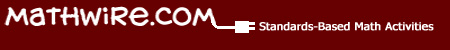Multiplication & Division Games## Featured Game:   Who Has? Multiplication Decks

Once students have developed conceptual understanding of the basic operations they need to develop fluency with the facts. One quick way to include daily practice and motivate students to master these basic facts is through the use of the Who Has? card decks. These decks can be created for virtually any topic and frequent use as both a whole class practice or as a center activity for partners or small groups will provide facts practice in a highly-motivating format.

• See Who Has? Activities for classroom management strategies for using the different multiplication decks, including one Spanish version.

### Multiplication Games

These games help students acquire proficiency in multiplication facts by requiring that students multiply factors to play the game.   Many of the games offer varying skill levels enabling the teacher to differentiate play levels to best match the varied needs of students in the class.• DownloadTriangle Tower Game which requires that students choose 10 products from the possible products created by tossing two regular dice and write one product in each square of the tower.   Each player tosses the two dice, calculates the product and covers that product on his/her game mat, if possible.   First player to cover all ten squares of his/her tower wins the game. Contributed by Maria Ricci, Joseph C. Caruso School, Keansburg, NJ
• Online directions for the Everyday Math gameMultiplication Bingo
• Play the Everyday Math gameFactor Bingo online.
• Online directions for the Everyday Math gameMultiplication Wrestling
• Play the Everyday Math gameBaseball Multiplication with 2 dice online.
•Target 300:   this Math Solutions game develops students' estimation skills as they choose to multiply each die toss by 10, 20, 30, 40, or 50 so that the sum of all six tosses will be the closest to 300.
•Closest to 1000:   This Math Solutions game challenges students' understanding of multiplication and helps develop estimation skills as they select appropriate starting numbers and multipliers to get closest to 1000 at the end of two multiplications.   Students use calculators for the multiplication problems as this game focuses on students' ability to think about multiplication and estimate the outcome of the double multiplication before using the calculator.
•One Time Only:   this Math Solutions game provides practice in identifying factors as students successively write down factors of the current number and try not to lose by being the person who cannot write a factor that has yet to be used in the game.
• PlayBatter's Up Baseball online to practice multiplication facts.
• See Games to Practice Multiplication Facts for a discussion of several of these games in more depth.

### Division Games

These games help students develop an understanding of division and remainders.

•Leftovers with 100:   this Marilyn Burns game challenges students to choose divisors to create the largest remainder since the remainder is the student's score for the round.   As with many good math games, students will ponder the choices and mentally calculate several possibilities in the search for the optimal divisor that yields the largest remainder and the most points.